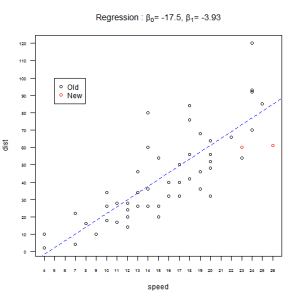Want to share your content on R-bloggers? click here if you have a blog, or here if you don't.Being able to visualize information through plots is essential for a statistic analysis. A simple and clean graph  can explain much more than words.  In this set of exercises you will test and learn advanced graphic arguments. Before you start check the documentation for the following functions: `plot``points`, `abline,` `title`, `legend` ,`par (`including all the arguments), `mfrow` and `layout`

For this set of exercises you will use the dataset called `cars`, an R dataset that contains two variables; distance and speed. To load the dataset run the following code line `data(speed)`.

Answers to the exercises are available here.

If you obtained a different (correct) answer than those listed on the solutions page, please feel free to post your answer as a comment on that page.

Exercise 1

a)Load the `cars` dataset and create a scatterplot of the data.

b)Using the argument `lab` of the function `plot` create a new scatterplot where the thickmarks of the x and y axis specify every integer.

Exercise 2

The previous plot didn’t showed all the numbers associated to the new thickmarks, so we are going to fix them. Recreate the same plot from the previous question and using the argument `cex.axis` control the size of the numbers associated to the axes thickmarks so they can be small enough to be visible.

Exercise 3

On the previous plot the numbers associated to the y-axis thickmarks aren’t easy to read. Recreate the plot from the last exercise and use the argument `las` to change the orientation of the labels from vertical to horizontal.

Exercise 4

Suppose you want to add two new observations to the previous plot, but you want to identify them on the graph. Using the `points` function add the new observations to the last plot using red to identify them. The values of the new observation are speed = 23, 26 and dist = 60, 61.

Exercise 5

As you could see the previous plot doesn’t show one of the new observations because is out the x-axis range.

a)Create again the plot for the old observations with an x-axis range that includes all the values from 4 to 26.

b)Add the two new observations using the `points` function.

Exercise 6

After running a linear regression to the original data you find out that a = 17.5 and b = 3.93. Using the function `lines` add the linear regression to the plot using blue and a dashed line.

Exercise 7

Using the function `title` and `expression` add the following title “Regression: β 0 = -17.3, β 1 = -3.93″.

Exercise 8

Add to the previous plot a legend on the top left corner that shows which color is assigned to old observations and which one to new ones.

Exercise 9

This exercise will test your skills to create more than one plot in the same layout. Using the functions `par` and `mfrow`.
Create on the same layout two histograms, one for each column of the `cars` data.

Exercise 10

Using the function `layout` print on the same layout 3 plots, on the left side a scatterplot of cars, on the top right the histogram of the column speed of the data `cars`, and on the bottom right an histogram of the column distance.